# Algorithm Visualizer "Algorithm Visualizer" which allows visual confirmation of code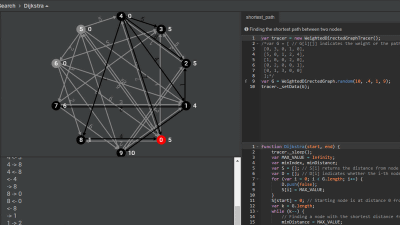When displaying algorithms programmatically, it is difficult to imagine a program of an algorithm to be executed because of the complex concept of the algorithm itself and abstract code. Program learning of such an abstract algorithm involves not only code, but also logs when actually running the program, while visualizing the algorithm "Algorithm VisualizerIt is very useful.

Algorithm Visualizer
http://jasonpark.me/AlgorithmVisualizer/

Algorithm Visualizer,Bubble sortYaBinary search (binary search)And other algorithms are displayed as a program while visualizing the movement in the case of actual execution or logging it so that you can deepen your understanding of the algorithm.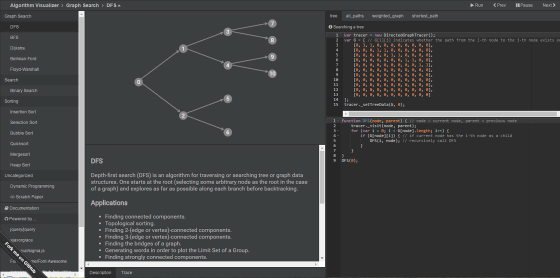The algorithm names are lined up on the left side of the page, and you can execute the program of the selected algorithm.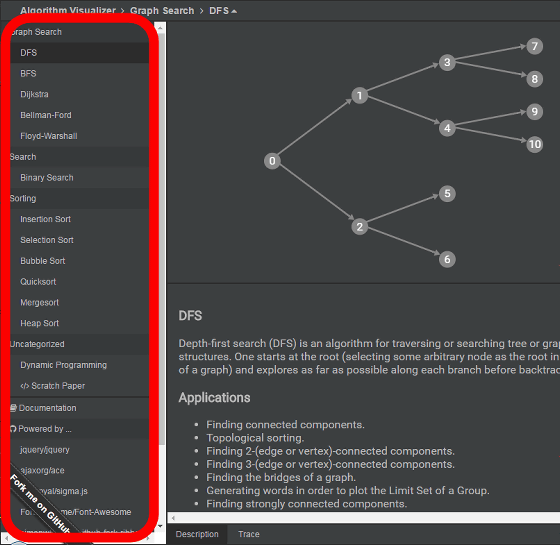At the top center of the page you can visualize the behavior of the algorithm you have run.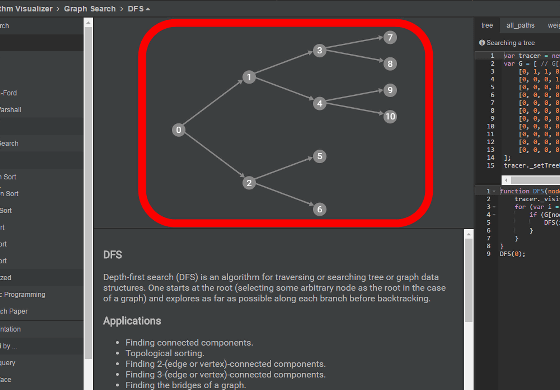At the upper right of the page, the program of initial setting (condition setting) of the algorithm to be executed is displayed ......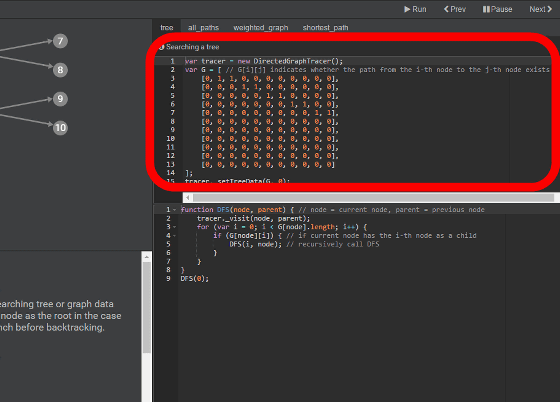Below that is a concrete program that implements the algorithm in Javascript.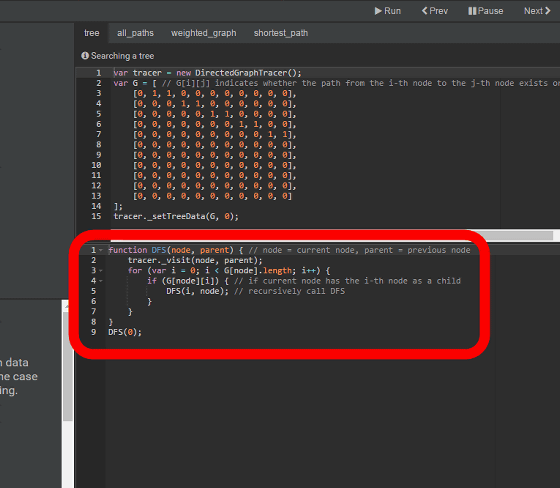Let's visualize the algorithm at once. Select "Bubble Sort" (Bubble Sort) and click "Run".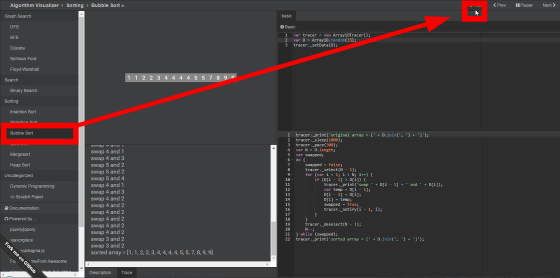Then, how the animation at the top of the page starts moving and the algorithm is executed will be visualized.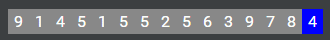In addition, under the animation, the progress of the algorithm was displayed as a log as described in the Javascript program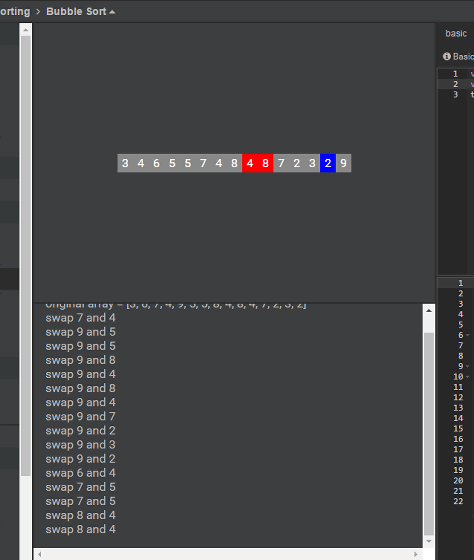The numerical value of the condition setting can be changed by keyboard input.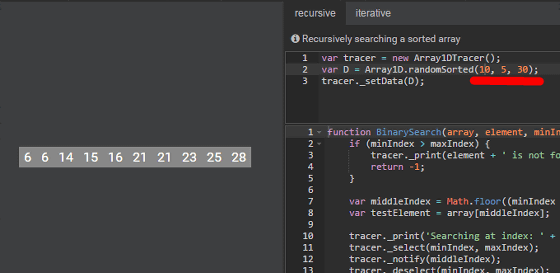You can also change specific programs regarding the algorithm.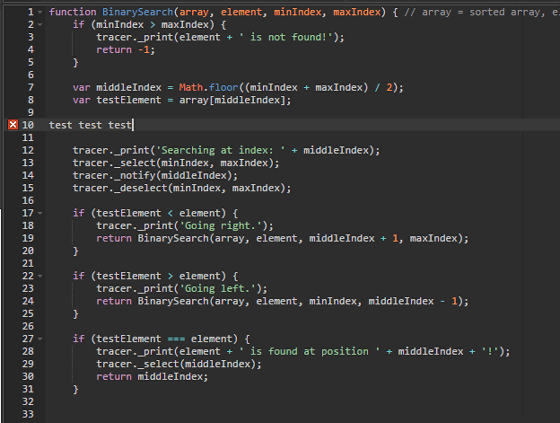At the time of writing the article Algorithm Visualizer's algorithm is like this.

· DFS (Depth-first search)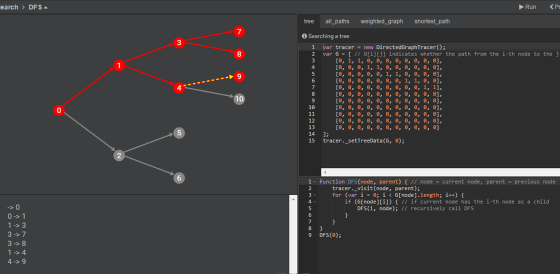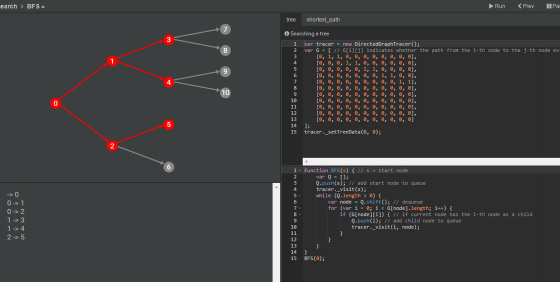· Dijkstra (Dijkstra method)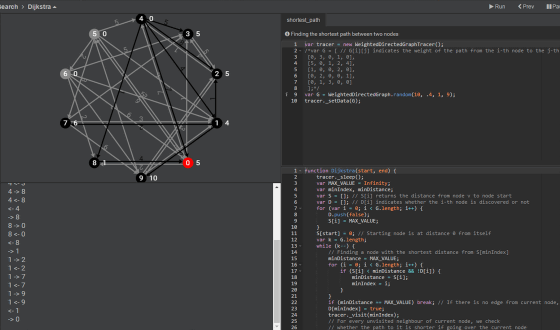· Bellman-Ford (Bellman-Ford method)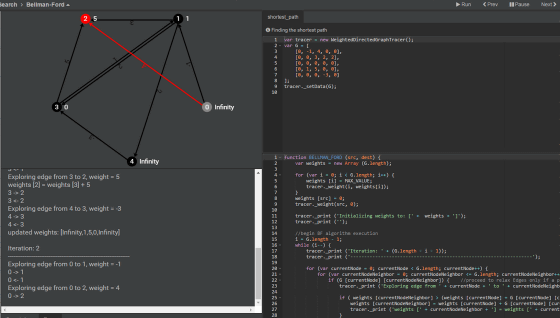· Warshall-Floyd (Warrant - Floyd method)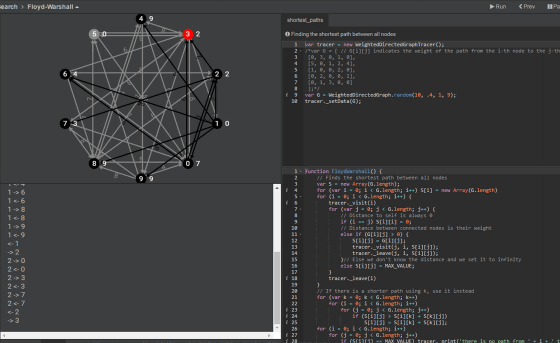· Insertion Sort (Insert sort)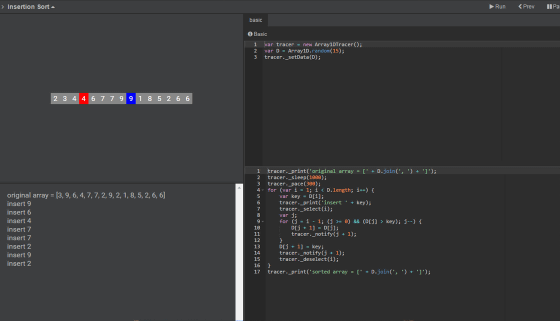· Selection Sort (Selective sort)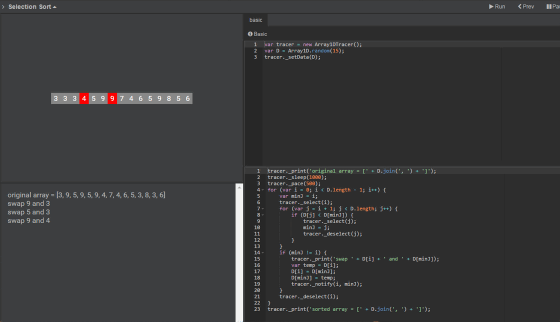· Bubble Sort (Bubble Sort)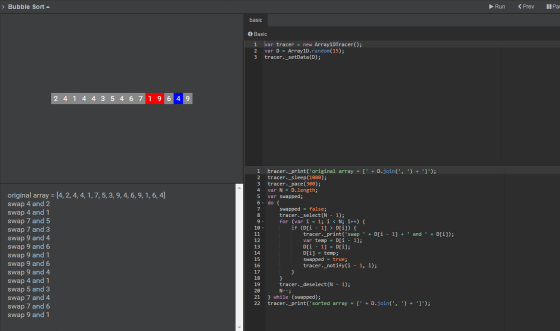· Quicksort (Quick sort)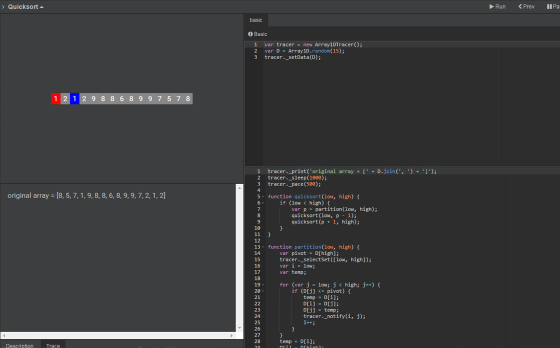· Mergesort (Merge Sort)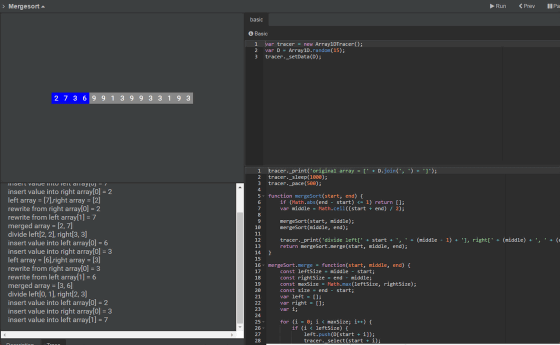· Heap Sort (Heap Sort)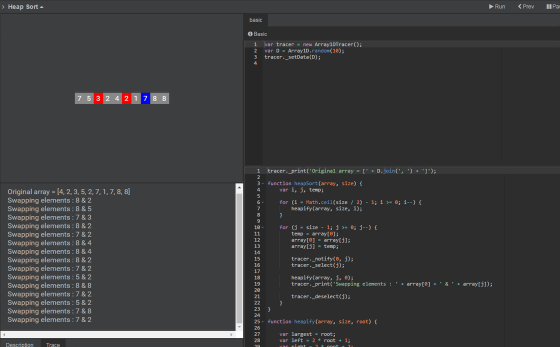Parkjs 814 created Algorithm Visualizer, and the program is open to the public at GitHub.

GitHub - parkjs814 / AlgorithmVisualizer: Algorithm Visualizer
https://github.com/parkjs814/AlgorithmVisualizer

Parkjs 814 calls on people who created programs of other algorithms to add data according to the published wiki on the above GitHub page.

in Web Service, Posted by darkhorse_log